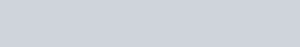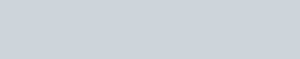# Re-arrange the data from columns to rows

​Transposing data in Excel is pretty straight forward. Just copy the data, goto Paste Special and check on the transpose box found in the Paste Special window. The data is immediately re-arranged with the dimensions switched, from rows to columns and columns to rows. But if you are planning to transpose partially so that you can use the data as a Pivot Table source, then the solution is not as straight forward. This happens when you are collating sales forecasts,

​budgets or working on payroll within Excel.​To do a partial transpose, you need to use the worksheet function INDEX.

1. In row 1, label all the columns from 1 to 13 starting from column B.

​2. In Column A, label the rows starting from 1 in row 2. Note the colours for the labels.​3. In Cell B11, enter the formula =INDEX(\$B\$2:\$P\$7,2,1). The INDEX formula will pick up the value within the specific range \$B\$2:\$P\$7, starting the row count from cell B2, as row 1 and column 1. The value "product 1" is displayed.

​4. To get the location in C3, the formula is =INDEX(\$B\$2:\$P\$7,2,2).

​5. Instead of manually entering the row and column into the INDEX formula, you can refer the row and column using the labels we have set up in Step 1 and 2 and in the following steps.

​6. Label the rows and columns as shown below.​7. The green range refers to the columns that need to be repeated for 12 months of records. The purpose range refers to the row to ber repeated. The pink range refers to the columns (months) that need to be transposed.

​8. The 2 INDEX formulas we have created initially can now be modified.​9. The month is in row 1 column 3. So the formula is =INDEX(\$B\$2:\$P\$7,1,\$J11).

​10. The amount can be picked up using the formula =INDEX(\$B\$2:\$P\$7,\$I11,\$J11).

​11. Once you have these formulas set up, you can copy the 4 formulas down to the rest of the 11 remaining rows.

​12. If you copy the formula down, the row number will change after every 12 rows. 13. Enter the formula = J11 into the cell J23. The formula will repeat the column numbering from above and reset the number every 12 rows. Copy the formulas to as many rows as required and use the data for Pivot Table.

12. To repeat the whole process, enter the formula =I11+1 into the cell I23. This will increase the row by one unit.